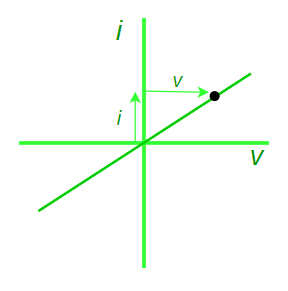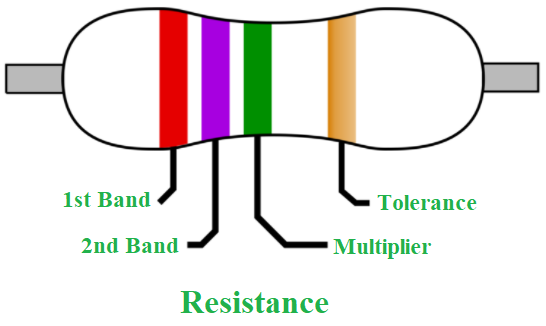# Ohm’s Law – Definition, Formula, Applications, Limitations

• Last Updated : 23 Nov, 2021

According to Ohm’s law, the voltage or potential difference between two locations is proportional to the current of electricity flowing through the resistance, and the resistance of the circuit is proportional to the current or electricity travelling through the resistance. V=IR is the formula for Ohm’s law. Georg Simon Ohm, a German physicist, discovered the connection between current, voltage, and relationship. Let’s take a closer look at Ohms Law, Resistance, and its applications.

### What is Ohm’s Law?

Voltage, current, and resistance are the three most fundamental components of electricity. Ohm’s law depicts a straightforward relationship between these three variables. According to Ohm’s law, the current flowing through a conductor between two locations is proportional to the voltage across the conductor.

Attention reader! All those who say programming isn't for kids, just haven't met the right mentors yet. Join the  Demo Class for First Step to Coding Course, specifically designed for students of class 8 to 12.

The students will get to learn more about the world of programming in these free classes which will definitely help them in making a wise career choice in the future.Voltage-Current Relationship Diagram

### Ohm’s Law Formula

This is one of the most fundamental electrical rules. It aids in the calculation of an element’s power, efficiency, current, voltage, and resistance in an electrical circuit.

V ∝ R

V = I × R

Here,

• V is the voltage,
• I is the current, and
• R is the resistance.

The SI unit of resistance is ohms and is denoted by Ω.

### Applications of Ohm’s Law

When the other two numbers are known, Ohm’s law may be used to determine the voltage, current, impedance, or resistance of a linear electric circuit.

Main applications of Ohm’s Law:

• It also simplifies power calculations.
• To keep the desired voltage drop between the electrical components, Ohm’s law is employed.
• An electric circuit’s voltage, resistance, or current must be determined.
• Ohm’s law is also utilised to redirect current in DC ammeters and other DC shunts.

### How do we establish a current-voltage relationship?

The ratio V ⁄ I remains constant for a given resistance while establishing the current-voltage connection, hence a graph of the potential difference (V) and current (I) must be a straight line.

How can we discover the unknown resistance values?

The constant ratio is what determines the unknown resistance values. The resistance of a wire with a uniform cross-section relies on the length (L) and the cross-section area (A). It also relies on the conductor’s temperature.

The resistance, at a given temperature,

R = ρ L ⁄ A

Here, ρ is the specific resistance or resistivity and is the wire material’s characteristic.

The wire material’s specific resistance or resistivity is,

ρ = R A ⁄ L

### Limitations of ohms law

• The law of Ohm does not apply to unilateral networks. The current can only flow in one direction in unilateral networks. Diodes, transistors, and other electronic components are used in these sorts of networks.
• Non-linear components are also exempt from Ohm’s law. Non-linear components have a current that is not proportional to the applied voltage, which implies that the resistance value of those elements varies depending on the voltage and current. The thyristor is an example of a non-linear element.

### Resistors

One of the most important components in electrical circuits is the resistor. Because they are comprised of a clay or carbon combination, they are good conductors and good insulators. Four colour bands are seen on the majority of resistors. The first and second bands show the value’s first and second digits, respectively. The value digits are multiplied in the third band, and the tolerance is determined in the fourth band. If there isn’t a fourth band, the tolerance is presumed to be plus or minus 20 %.Resistance in series

A series is a group of related items, such as along a line, in a row, or in a certain order. In electronics, series resistance implies that the resistors are linked in series and that current can only travel via one channel.

Laws of Series Circuits

• The overall circuit resistance is made up of individual resistances.
• The total voltage is the sum of the individual voltages in the circuit.
• Every point in the circuit has the same amount of current flowing through it.

Resistance in parallel

A parallel circuit can be organised in a variety of ways. The majority of wiring in the real world is done in parallel, such that the voltage provided to any one portion of the network is the same as the voltage given to any other section of it.

Laws of Parallel Circuits

• All of the component resistances’ reciprocals add up to the overall circuit resistance’s reciprocal.
• The total current draw is the sum of individual current pulls across the circuit.
• Every point in the circuit has the same voltage.

### Sample Problems

Problem 1: Find the resistance of an electrical circuit with a voltage supply of 15 V and a current of 3 mA.

Solution:

Given:

V = 15 V,

I = 3 mA = 0.003 A

The resistance of an electrical circuit is given as:

R = V / I

= 15 V / 0.003 A

= 5000 Ω

= 5 kΩ

Hence, the resistance of an electrical circuit is 5 kΩ.

Problem 2: If the resistance of an electric iron is 10 Ω and a current of 6 A flows through the resistance. Find the voltage between two points.

Solution:

Given:

I = 6 A

R = 10 Ω

The formula to calculate the voltage is given as:

V = I × R

V = 6 A × 10 Ω

= 60 V

Hence, the voltage between two points is 60 V.

Problem 3: When does Ohm’s law fail?

Solution:

The behaviour of semiconductors and unilateral devices like diodes defines Ohm’s law. If physical factors such as temperature and pressure are not kept constant, Ohm’s law may not provide the intended effects.

Problem 4: Why doesn’t Ohm’s law apply to semiconductors?

Solution:

Semiconducting devices are nonlinear in nature due to which Ohm’s law does not apply to them. This indicates that the voltage-to-current ratio does not remain constant when voltage varies.

Problem 5: What is the application of Ohm’s Law?

Solution:

The static values of circuit components such as current levels, voltage supplies, and voltage drops are validated using Ohm’s law.

My Personal Notes arrow_drop_up